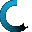Retrieving data ...
Please do not click anywhere else.

If you see no response after 2-3 minutes, then click on the [X] button in the upper right corner to close the window and retry the operation at a later time.
Interactive Multimedia Learning & Teaching Management System Suite## Course Contents

Course Name: Elementary Algebra - Online
• Elementary Algebra
•  1.1 Recognizing and Predicting Patterns
•  1.2 Extending Patterns
•  1.3 Increasing Number Patterns
•  1.4 Decreasing Number Patterns
•  1.5 Balancing Equations
•  1.6 Arithmetic Number Patterns
•  1.7 Geometric Number Patterns
•  1.8 Find the Missing Number
•  1.9 Properties of Addition and Multiplication
•  1.10 Completing Equations
•  1.11 Numeric Expressions and Equations
•  1.12 Number Comparisons using Symbols
•  1.13 Completing Number Sentences with Addition
•  1.14 Comparing Number Expressions with Addition
•  1.15 Completing Number Sentences with Subtraction
•  1.16 Comparing Number Expressions with Subtraction
•  1.17 Number Patterns using Addition and Subtraction
•  1.18 Number Patterns using Multiplication and Division
•  1.19 Unknown Variables in Mathematical Equations
•  1.20 The Number Line
•  1.21 Variables, Expressions and Equations
•  1.22 Writing Expressions and Equations
•  1.23 Inequalities using Variables
•  1.24 Formulas
•  1.25 Order of Operations
•  1.26 Evaluating Expressions
•  1.27 Equations with One Variable